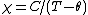# Curie-Weiss Law

Also found in: Dictionary, Wikipedia.

## Curie-Weiss law

A relation between magnetic or electric susceptibilities and the absolute temperature which is followed by ferromagnets, antiferromagnets, nonpolar ferroelectrics and antiferroelectrics, and some paramagnets. The Curie-Weiss law is usually written as the equation below,where χ is the susceptibility, C is a constant for each material, T is the absolute temperature, and Θ is called the Curie temperature. Antiferromagnets and antiferroelectrics have a negative Curie temperature. The Curie-Weiss law refers to magnetic and electric behavior above the transition temperature of the material in question. It is not always precisely followed, and it breaks down in the region very close to the transition temperature. Often the susceptibility will behave according to a Curie-Weiss law in different temperature ranges with different values of C and Θ. See Curie temperature, Electric susceptibility, Magnetic susceptibility

McGraw-Hill Concise Encyclopedia of Physics. © 2002 by The McGraw-Hill Companies, Inc.
The following article is from The Great Soviet Encyclopedia (1979). It might be outdated or ideologically biased.

## Curie-Weiss Law

a temperature dependence of the specific magnetic susceptibility Χ of paramagnetic materials having the form

(1) Χ = C′/(T -Δ)

where C′ and Δ are constants for a given substance (P. Weiss, 1907). Equation (1) describes the experimental dependence of Χ on the temperature T with sufficient accuracy for most cases of paramagnetism of ions in crystals, as well as in crystals possessing atomic magnetic ordering at T > Ⓗ (above the Curie and Néel points). In many cases, the constant C ′, in practice, coincides with the constant C of Curie’s law for magnetic ions of a given type. The constant Δ characterizes the interaction of the magnetic ions with one another and with the crystal lattice field.

The magnetic susceptibility of paramagnetic substances, which become ferromagnetic at low temperatures, is described by equation (1) with a positive value of Δ close to the value of the Curie temperature Ⓗ. For substances that undergo a transition to an antiferromagnetic state at low temperatures, in most cases Δ is negative and agrees with the Néel temperature TN only in order of magnitude.

The Curie-Weiss law is also applicable to ferroelectrics. At temperatures T , >> Ⓗ (where Ⓗ is the Curie temperature of the ferroelectric), the dielectric constant is ∊ = B/(T — Ⓗ), where B is a constant for the substance.

## Curie-Weiss law

[¦kyu̇r·ē ¦vīs ‚lȯ]
(electromagnetism)
A relation between magnetic or electric susceptibilities and the absolute temperatures which is followed by ferromagnets, antiferromagnets, nonpolar ferroelectrics, antiferroelectrics, and some paramagnets.
McGraw-Hill Dictionary of Scientific & Technical Terms, 6E, Copyright © 2003 by The McGraw-Hill Companies, Inc.
References in periodicals archive ?
has been shown to be valid over a wide temperature range instead of the normal Curie-Weiss law (11) [27, 28].
1/([chi] - [[chi].sub.0]) value for a-Ce[Ru.sub.2] [Al.sub.10] alloy exhibits a linear behavior in the high- temperature region of T > 30 K following the Curie-Weiss law,
A fit to the Curie-Weiss law above 120 K results in a paramagnetic Curie temperature [[theta].sub.p] ~ -35 K and the effective moment [[mu].sub.eff] ~ 5.5 [[mu].sub.B], which is somewhat bigger than the expected free [Co.sup.2+] ion value with spin S = 3/2.

Site: Follow: Share:
Open / Close